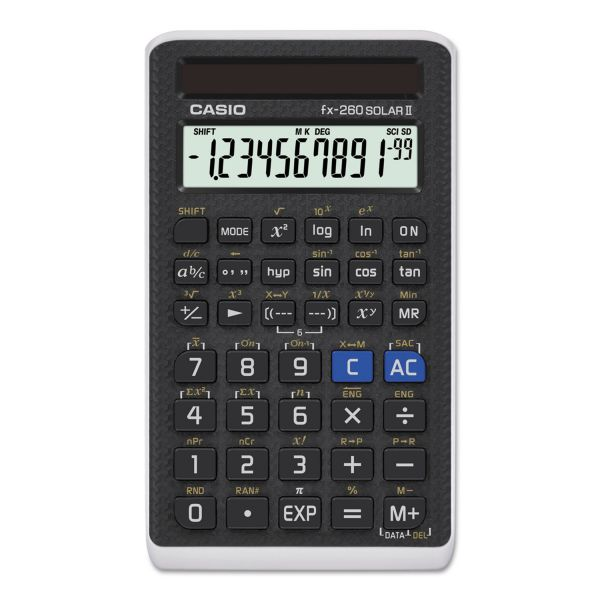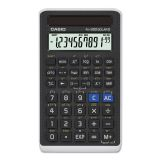# Casio FX-260 Solar All-Purpose Scientific Calculator, 12-Digit LCD

Product Number: CSOFX260SLRII
• All-purpose scientific calculator.
• Offers fraction calculation and trigonometric functions.
• Protects memory.
More details
\$11.43
Free Shipping Orders Over \$45+ Estimated Delivery
Favorite
Compare
Request Quote

## Product Description

Casio FX-260 Solar All-Purpose Scientific Calculator, 12-Digit LCD - All-purpose scientific calculator offers fraction calculation, trigonometric functions and is solar powered.

• All-purpose scientific calculator.
• Offers fraction calculation and trigonometric functions.
• Protects memory.### Product Specifications

• +/- Switch Key
Yes
• Amortization
No
• Assembly Required
No
• Backspace Key
Yes
• Base Number Calculations
Yes
• Bond Calculations
No
• Brand Name
Casio
• Case
Hard
• Cash Flow Calculations
No
• Complex Number Calculations
No
• Confidence Interval Calculating
No
• Cost/Sell/Margin
No
• Country of Origin
TH
• Currency Exchange Function
No
• Date Calculations
No
• Decimal Function
Yes
• Depreciation Calculations
No
• Display Angle
Fixed
• Display Characters x Display Lines
10 x 1
• Display Notation
Numeric
• Display Type(s)
LCD
• Double Zero Key
No
• Entry Logic
No
• Equation Editor
No
• Fraction Calculations
Yes
• Fraction/Decimal Conversions
Yes
• Global Product Type
Calculators-Scientific
• Grand Total Key
No
• Graphing Functions
No
• Higher Mathematical Functions
Statistics Trigonometry
• Hyperbolic Functions
Yes
• Hypothesis Testing
No
• Interest Rate Conversion
No
• Item Count Function
No
• Levels of Parentheses
4
• Linear Regression
No
• Loan Calculation
No
• Logical (Boolean) Operations
No
• Markup/Down Key
No
• Matrices
No
• Memory
Independent
• Metric Conversion
No
• Number of Display Digits
12
No
• Percent Key(s)
Yes
• Polar-Rectangular Conversion
Yes
• Post-Consumer Recycled Content Percent
0%
• Power Source(s)
Solar
• Pre-Consumer Recycled Content Percent
0%
• Probability (Random Number)
Yes
• Programming Steps
No
• Recycled
No
• Simultaneous Equations
No
• Size
2.75 x 4.75
• Square Root Key
Yes
• Tax Calculation
No
• Time-Value-of-Money
No
• Time/Date
No
• Total Recycled Content Percent
0%
• Trig/Log Functions
Yes
• Variable Regression
No
• Variable Statistics
Yes